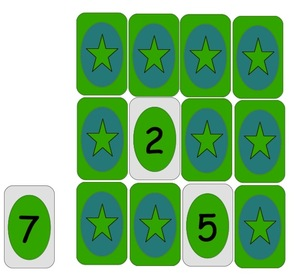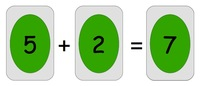# Kiri's Mathematics Match Game

Alignments to Content Standards: 1.OA.D.8

• Students can play in groups of 2-4.
• An array of cards (twelve to twenty in total) is placed face down and one card, called the target card, is put face up.
• The students take turns flipping over two cards, one at a time.
• If the sum or difference of the values on the two cards equals the value on the target card, the student who exposed those cards should say a number sentence to express the relationship. If they are correct, the three cards are removed and replaced so there is again a full array.
• If a student does not combine the values of flipped cards to make the value on the target card, then it is the next student's turn.
• In the no-memory-needed version of the game, all chosen cards are left face up (after an unsuccessful turn) and may be used to make matches. In the light-memory version, cards are left face up until there is a match, after which all are put face down. In the memory version, cards are put face down after an unsuccessful turn before the next player's turn.## IM Commentary

• In all versions, students must engage basic addition and subtraction facts. In the memory version, after a student has turned over one card, in order to know whether there is a match using cards they've seen, they need to to solve equations of the form $\square+b=c$, $b + \square=c$, $\square-b=c$, and $b-\square=c$.

• Students could also be asked to record the number sentences they make, either with available "symbol" cards as envisioned in the hypothetical picture above, or on paper or white board to reinforce written work and allow the teacher to evaluate whether students are making all possible kinds of number sentences.

• Teachers could make cards, or have students make them, or use numbered cards from a standard deck or by taking cards from other games. Zeros would be appropriate, and ''wilds'' could also naturally be incorporated. The target card values should be up to $20$ to fully meet the standard (with target cards kept separately).

• To extend, and incorporate 1.OA.7 into this activity, there could be two target cards to match in total or difference and/or students could flip over three cards and possibly use all of them.

• Note: This game was invented by Kiri, a first grader.

## Solution

Students fully meet the mathematical demands of the no-memory version if they can perform all appropriate addition and subtractions with the target card and exposed cards to check whether there are any matches. Using the inverse relationship between addition and subtraction should be encouraged. For example, if the target card is $3$ and one of the exposed cards is $5$ the students might want to look for any $8$'s exposed, as they recognized that because $3 + 5 = 8$, they will be able to make the number sentence $8 - 5 = 3$.

For the versions which involve memory, the mathematical demands are met if after they flip over one card they can name all possible second cards which could be used to complete a valid number sentence and/or evaluate the possibility of using previously flipped cards which they remember. For example, if the target is $7$, and the first student turns over a $2$ and an $8$, then if the second student turns over a $1$ they should want to recall the location of the $8$ in order to make the number sentence $8 - 1 = 7$.